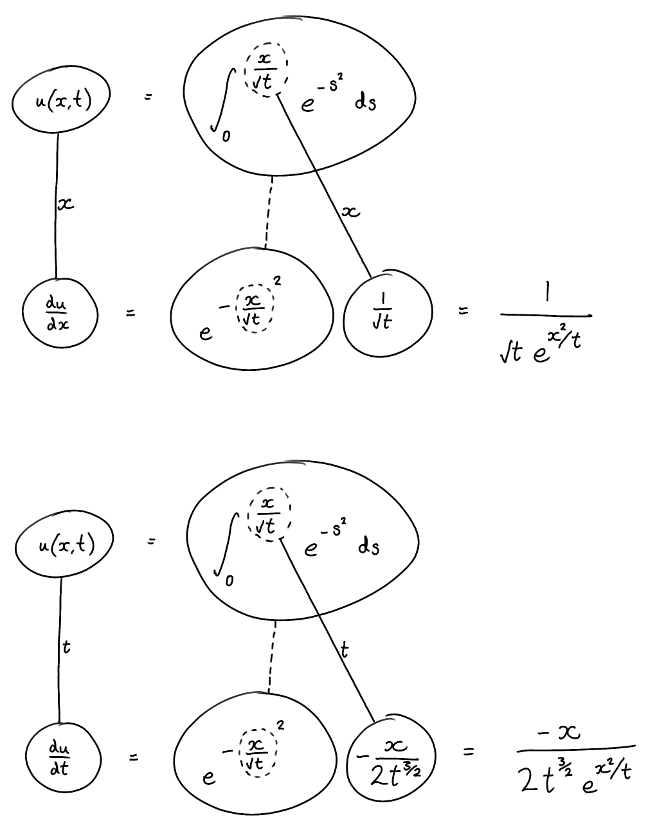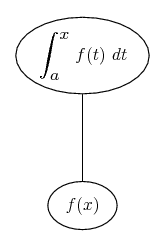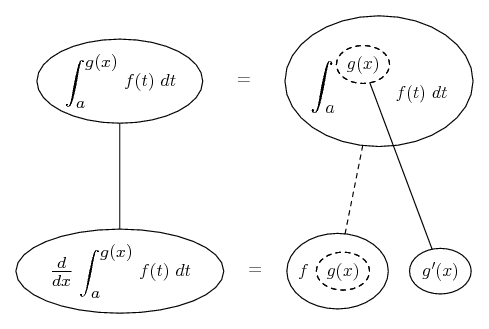# Thread: Show that u(x,t) satisfies this partial differential equation

1. ## Show that u(x,t) satisfies this partial differential equation

For $\displaystyle x$ $\displaystyle \in$ $\displaystyle R$ and $\displaystyle t > 0$ , define

$\displaystyle u(x, t) :=$ $\displaystyle \int_0^{x/\sqrt{t}} \! e^{-s^2} \, \mathrm{d}s$
Show that u satisfies the partial differential equation $\displaystyle 4{du/dt} = d^2u/dx^2$

2.Originally Posted by maximus101For $\displaystyle x$ $\displaystyle \in$ $\displaystyle R$ and $\displaystyle t > 0$ , define

$\displaystyle u(x, t) :=$ $\displaystyle \int_0^{x/\sqrt{t}} \! e^{-s^2} \, \mathrm{d}s$
Show that u satisfies the partial differential equation $\displaystyle 4{du/dt} = d^2u/dx^2$
Use the Fundamental theorem of Calculus and the chain rule.

Here is the first one

$\displaystyle \displaystyle \frac{\partial u}{\partial t}=e^{-\left( \frac{x}{\sqrt{t}}\right)^2}\cdot \frac{\partial }{\partial t}\left( \frac{x}{\sqrt{t}}\right)=-\frac{3}{2}\left( \frac{x}{t^{\frac{3}{2}}}\right)e^{-\frac{x^2}{t}}$

3. Just in case a picture helps...... where (key in spoiler) ...

Spoiler:... is the chain rule. Straight continuous lines differentiate downwards (integrate up) with respect to the main variable (in this case either x or t), and the straight dashed line similarly but with respect to the dashed balloon expression (the inner function of the composite which is subject to the chain rule).

Then, combining this with...... the Fundamental Theorem of Calculus, we have..._________________________________________

Don't integrate - balloontegrate!

Balloon Calculus; standard integrals, derivatives and methods

Balloon Calculus Drawing with LaTeX and Asymptote!

#### Search Tags

differential, equation, partial, satisfies